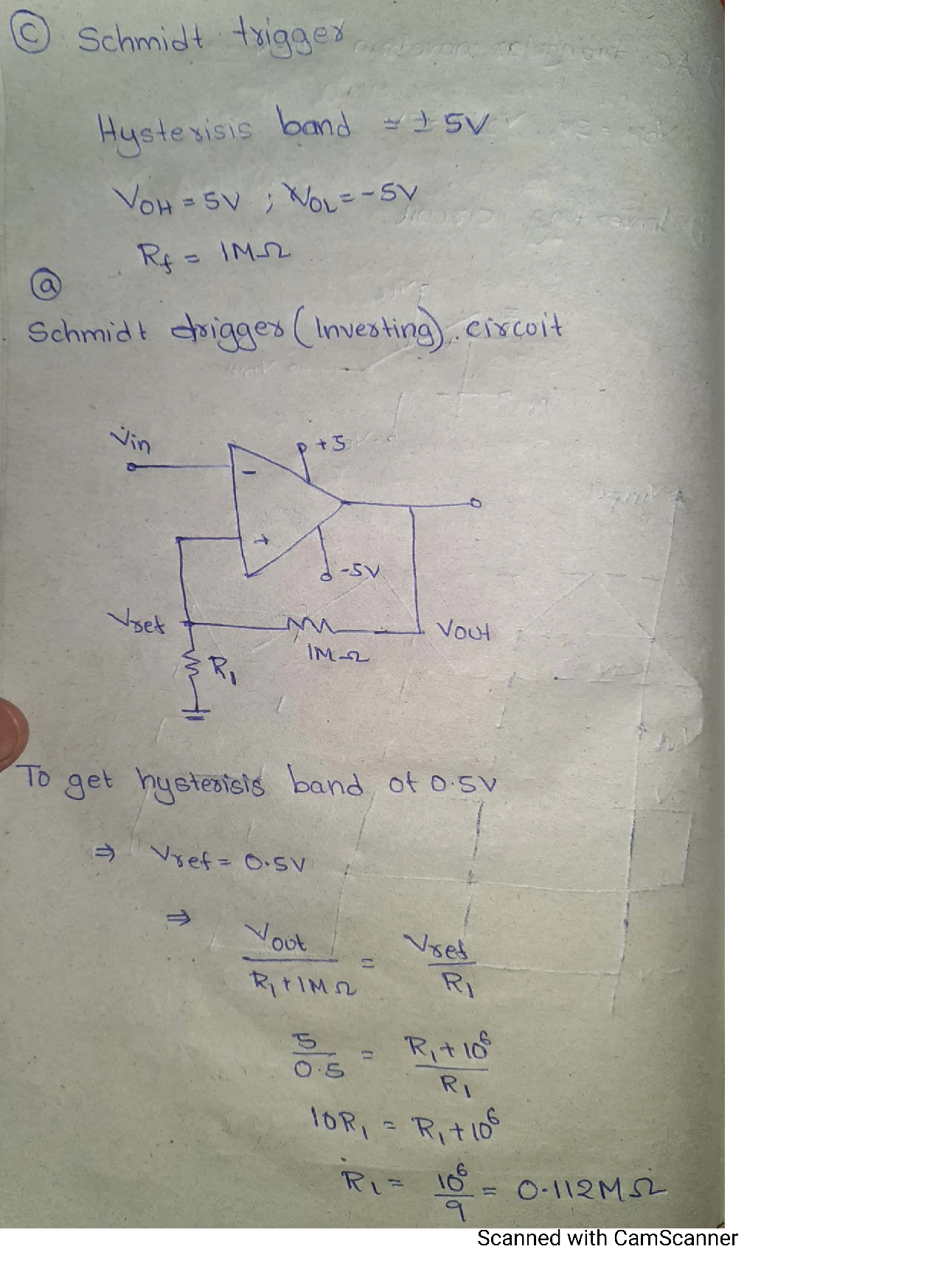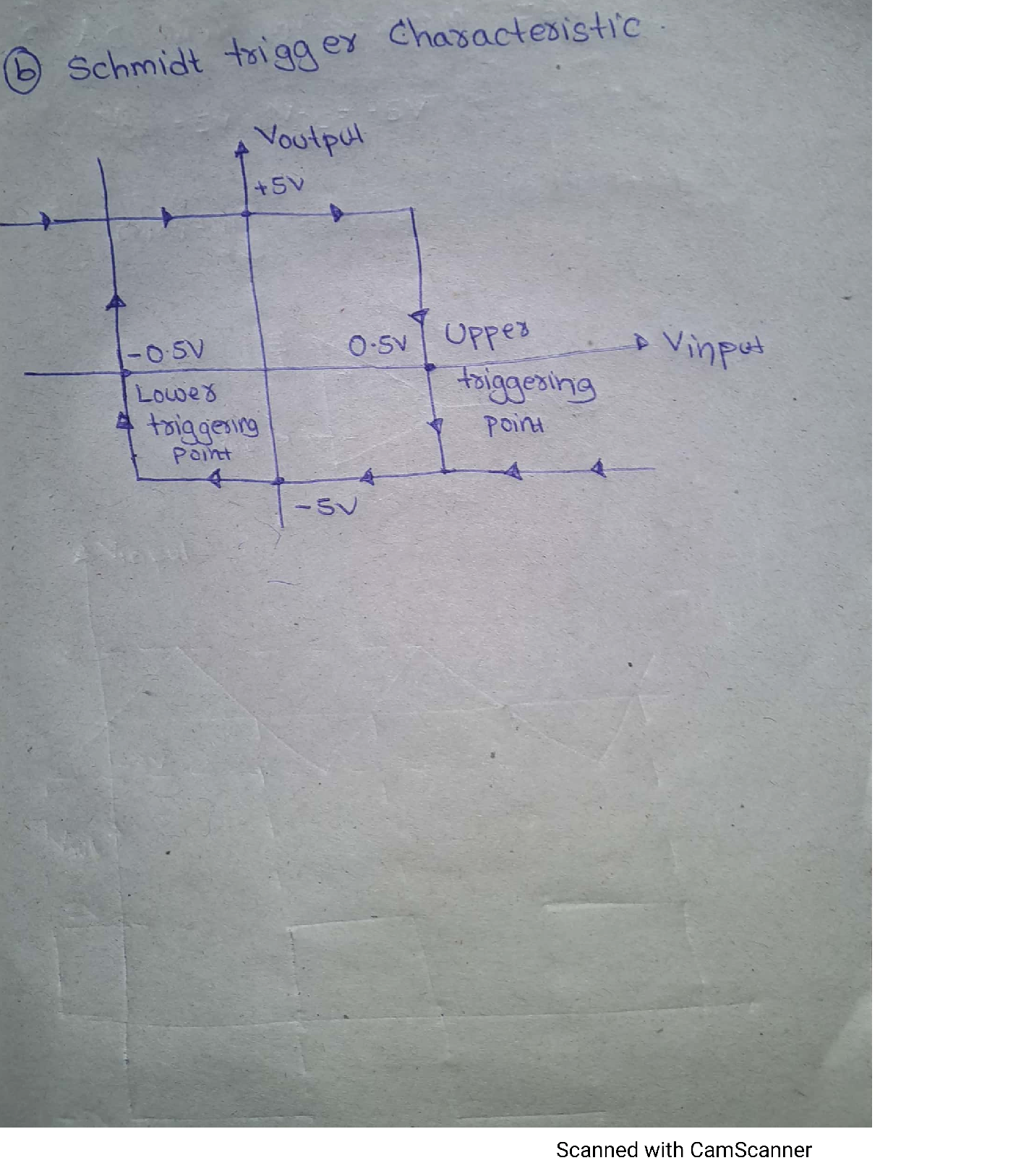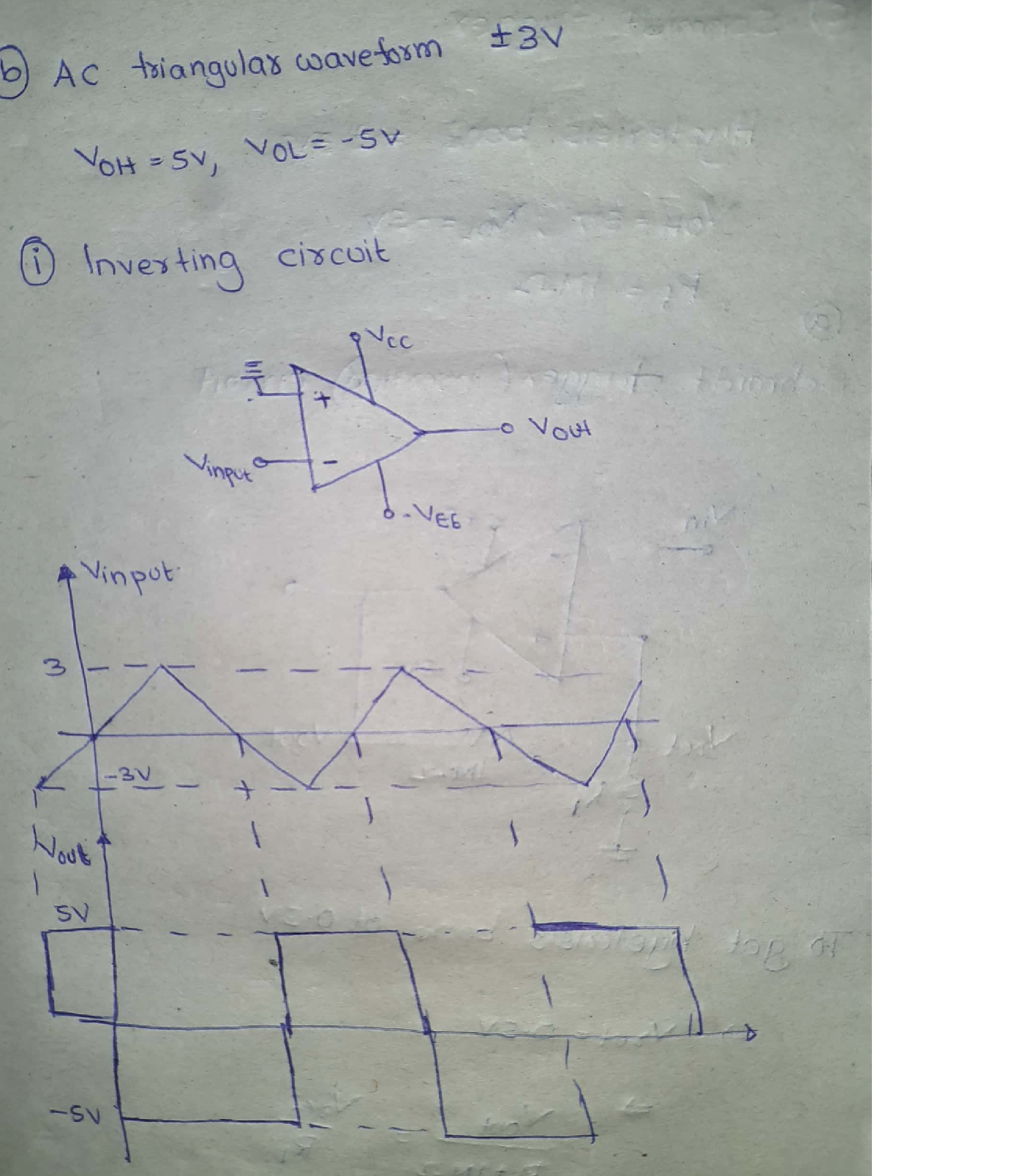Question

Basic Electronics

) Design a Schmitt trigger with a hysteresis band of±0.5V. The outputs of the Schmitt trigger areVOH=5V, VOL=-5V. The resistance value of the resistor in the positive feedback is 1MQ.

a) Explain the main limitations of an operational amplifier to be used as a comparator.

b) An AC triangular waveform with peak values of+3V is used as an input to a comparator. The outputs of the comparator are VoH= 5V, VOL= - 5V

Draw a waveform of the output of the(i)comparator when an inverting circuit isused.(5 Marks)

(ii)comparator when an inverting circuit withan offset of +2V is used.Draw a waveform of the output of the

c) Design a Schmitt trigger with a hysteresis band of±0.5V. The outputs of the Schmitt trigger areVoH=5V, VOL=-5V. The resistance value of the resistor in the positive feedback is 1MQ.

Draw the circuit diagram of a Schmitt trigger of an inverting circuit and determine the resistance of the resistor connected to the input.i)

ii)Draw the hysteresis characteristic of the Schmitt trigger indicating Upper Triggering point, Lower triggering Point,saturate voltages, input voltage andoutput voltage.(5Verified### Question 44843Basic Electronics

4a. Based on Fig. 4a, explain the fundamental working principle of a PN LED.
b. Fig. 4b shows phosphorus and boron doping of silicon. Using the diagram,explain how doping is achieved, the type of doping and the role of electrons and holes in the resultant material.
c. State the Stefan-Boltzmann law, explain its parameters and how it could be used as a basis for a passive infra-red (PIR) thermal imaging system.

### Question 44842Basic Electronics

OExplain what is the main focus of the branch of science known as"Metrology' and how it may be related to sensors.
(b) The terms accuracy, precision, resolution and linearity are clearly defined in Metrology. Explain these terms, giving examples or-sketching diagrams to highlight key features.

### Question 44841Basic Electronics

(a) Explain what is a low pass filter. Sketch a circuit diagram of apassive low pass filter and its bode plot for gain and phase. Explainthe significance of the -3dB point and what would the roll-off rate befor a 3rd-order low pass filter.
(b) Contrast the low pass filter with a high-pass filter. Sketch a circuit diagram of a passive high pass filter and its bode plot for gain and phase.[10 Markel
(c) What is the main difference between an active and a passive filter and contrast at least one key feature of an analogue Bessel filter versus an analogue Butterworth filter.

### Question 44840Basic Electronics

1.(a) Many sensors have a number of key characteristics in common,such as sensitivity and stability. List a further set of 5 key characteristics and explain why they are important.
(b) Explain what type of sensor is a silicon photodiode and its operation.What are the two main configurations of a photodiode and which of these is useful if the response of the photodiode needs to be relatively fast compared to a conventional photodiode.40 Modike)

### Question 44748Basic Electronics

The partial ac equivalent circuit of an amplifier is shown below. If R1 = 38 kohms and R2 = 1.99 kohms, find the overall voltage gain Vo/Vg (approximately)?

### Question 44747Basic Electronics

Which of the following is the best estimate for the resistance seen by the source, Rin, and the outpu tresistance seen by the load, Ro, for the circuit shownbelow?
O 10 x R1, 1 m2
O R1, 1N
O 10 x R2, 100 ohms
R2, 4.7 kohms

### Question 44746Basic Electronics

The amplifier shown below is very close to being an ideal trans resistance amplifier with a gain of 4.7 kohms.If Rg is 1.6 k2 and RL is 2.0 kohms, calculate the overall gain Vo/Vg.

### Question 44745Basic Electronics

The open loop gain, Aol of an amplifier with a feedback fraction of B =0.53, changes from 100 to110 because of temperature changes (hint: a large change!). Calculate the percentage change in the closed loop gain, Af. (Don't put the % sign in the answer).Type your answer...

### Question 44744Basic Electronics

The Transr esistance gain of an amplifier is defined as the ratio of
O The input voltage to the output current
The output current to the input voltage
O The output voltage to the input voltage
O The feedback current to the output voltage
O The output voltage to the input current

### Question 44743Basic Electronics

Estimate the magnitude of the loop gain of the circuit shown below. The op-amp has the following parameters: differential input resistance, rd = 1 MQ,output resistance, ro = 100 ohms open-loop gain, Aol-105 assume R1= 98.30 k ohms and R2 6.90 k ohms

### Submit query